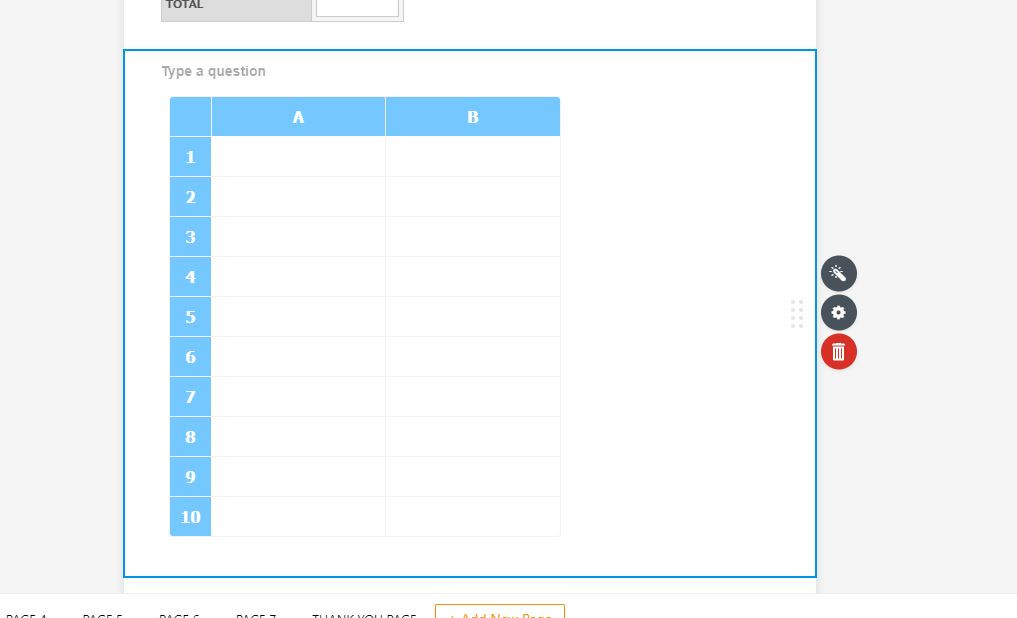How can I create a table that automatically populates total amount?

•CollectiveWellnessGroup
Asked on September 12, 2019 at 01:53 AM

Hi,

I would like to create a table where clients can input dollar figures, I would like the bottom of the spreadsheet to have a formula to create a total automatically so they do not have to manually add the total amount. I would like this cell to be locked so that they can not change the total.

I have had a look at the Spreadsheet and Spreadsheet Form and having issues. Is the best thing to import a spreadsheet that already has the formula? Can they then edit it on the form?

Chrissie

Page URL:
https://www.jotform.com/build/92537583127867•AndrewHag
Answered on September 12, 2019 at 02:50 AM

You can use the Spreadsheet widget to calculate the total value automatically. Also, the user can edit the values and the total will be calculated based on the new values. And you can also make the field read-only so the user cannot edit them.

It seems you already added the widget and created a formula to calculate total in b10. The formula is correct, but a small change would be, please write 'b10' instead of 'B10' and add a space after 'b10'.

Here is the correct format: b10 =b1+b2+b3+b4+b5+b6+b7+b8+b9

And to make the field read-only, you just need to write the cell number in the Read-Only tab.